# GMAT Math : DSQ: Calculating the length of an edge of a tetrahedron

## Example Questions

### Example Question #1 : Rectangular Solids & Cylinders

Find the length of the edge of a tetrahedron.

Statement 1: The volume is 6.

Statement 2: The surface area is 6.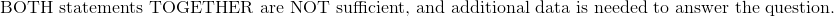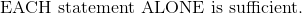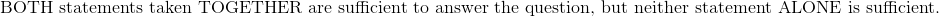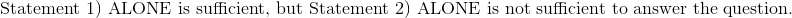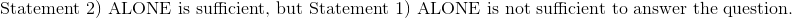Explanation:

Statement 1:) The volume is 6.

Write the formula to find the edge of the tetrahedron given the volume.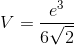Given the volume, it is possible to find the edge of the tetrahedron.

Statement 2:) The surface area is 6.

Write the formula to find the edge of the tetrahedron given the surface.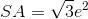Substitute the surface area to find the edge.

Therefore: